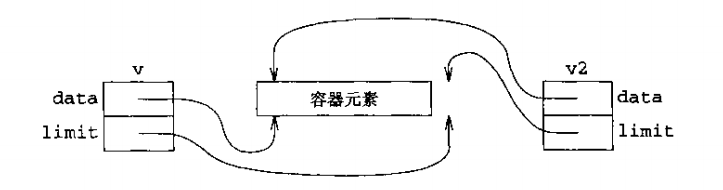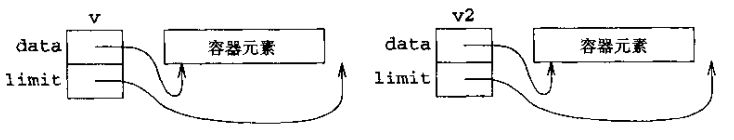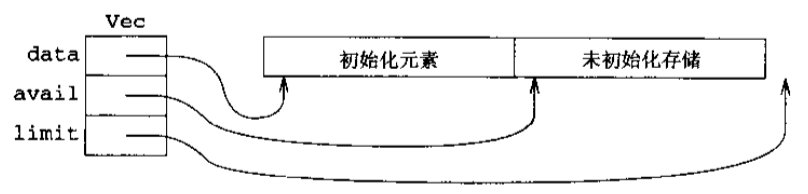# Accelerated C++

## 第1章 使用字符串

### 输入字符串

``````string name;
cout << "name:";
cin >> name;
cout << "hello" << name << endl;``````

## 第3章 使用批量数据

``````double x;
vector<double> homework;
while( cin >> x )
homework.push_back(x);

sort( homework.begin(), homework.end() ); // 排序成非递减序列

auto mid = homework.size() / 2;
median = homework.size() % 2 == 0 ? (homework[mid] + homework[mid-1])/2 : homework[mid]; // 中位数``````

## 第4章 组织程序和数据

``````// 计算中位数
double median( vector<double> vec )
{
auto size = vec.size();
if( vec.empty() )
throw domain_error( "median of an empty vector" );
sort( vec.begin(), vec.end() );
auto mid = size / 2;
return ( size % 2 == 0 ) ? ( vec[mid] + vec[ mid - 1 ] ) / 2 : vec[ mid ];
}

// 将输入流中家庭作业的成绩读入到vector中
istream &read_hw( istream &in, vector<double> &hw )
{
if( in )
{
hw.clear(); // 清除vector原来内容
double x;
while( in >> x )
hw.push_back(x);
in.clear(); // 清除错误标记，以使输入动作对下一个学生有效
}
return in;
}``````

``````// 把一个学生所有数据放一起
struct Student_info{
std::string name;
double midterm_score;
double final_score;
std::vector<double> homework;
};

// 读入一个学生的信息
istream &read( istream &is, Student_info &s )
{
is >> s.name >> s.midterm_score >> s.final_score;
return is;
}

// 比较名字 按引用传递
bool comp_name(const Student_info &x, const Student_info &y)
{
return x.name < y.name;
}``````

## 第5章 使用顺序容器并分析字符串

`vector`里依次存入多个学生信息：

``````vector<Student_info> students;
Student_info temp;
while( read( cin, temp ) )
{
students.push_back(temp);
}``````

``````// 计算最终成绩
double grade( double m_score, double f_score, double h_score )
{
return 0.2 * m_score + 0.4 * f_score + 0.4 * h_score;
}
double grade( double m_score, double f_score, const vector<double> &hw )
{
if( hw.empty() )
throw domain_error("no homework");
return grade( m_score, f_score, median( hw ) );
}
double grade( const Student_info &s )
{
return grade( s.midterm_score, s.final_score, s.homework );
}

// 判定不及格
bool fgrade( const Student_info &s )
{
return grade( s ) < 60;
}

// 从 students 中去除不及格的学生，同时返回它们
vector<Student_info> extract_fails( vector<Student_info> &students )
{
vector<Student_info> fail;
auto iter = students.begin();

while( iter != students.end() )
{
{
fail.push_back(*iter);
// 进行 erase 操作后，所有位于删除元素后面的元素的迭代器都会失效。
// 所幸，erase 返回了一个迭代器，它指向我们刚刚删除的元素的后一个元素
// 所以赋值给 iter 后，迭代器继续生效
iter = students.erase(iter);
}
else
++iter;
}
return fail;
}``````

## 第6章 使用库算法

``````// 对容器 c 产生一个迭代器，用于从尾部添加元素，要求容器要支持 push_back() 操作
back_inserter( c );

// 对容器 c 产生一个迭代器，用于从头部添加元素，要求容器支持 push_front() 操作
front_inserter( c );

// 判断两个序列是否相等，equal 假定第二个序列与第一个序列长度相等
equal( c1.begin(), c1.end(), c2.begin() );

// 从序列中查找值
find( c.begin(), c.end(), value );

// 查找符合 func 条件的元素，返回该元素的迭代器
find_if( c.begin(), c.end(), func );

// 查找 序列2 在 序列1 中的位置，如果没有找到，则返回 c1.end()
search( c1.begin(), c1.end(), c2.begin(), c2.end() );

// 使用 func 处理 c1 序列的每个元素，并且将结果添加在 c2 序列的后面
transform( c1.begin(), c1.end(), back_inserter(c2), func );

// 复制bottom中所有元素，添加到 ret 的末尾
copy( bottom.begin(), bottom.end(), back_inserter(ret) );

remove( b, e, v )               // [b,e) 删 value
remove_if( b, e, func )         // [b,e) 删 func 条件
remove_copy( b, e, r, v )       // [b,e) 删 v，结果存入 r
remove_copy_if( b, e, r, func ) // [b,e) 删 func 条件，结果存入 r

// 累加求和, 从初始值42开始，将 vec 中的各元素累加，它的返回值类型就是初始值的类型
accumulate( vec.begin() , vec.end() , 42 );
// 从空字符串开始，将 vec_str 里每一个元素链接成一个字符串
accumulate( vec_str.begin(), vec_str.end(), string(" "));

// 分组，将序列里的元素按条件 func 分为两部分，返回指向第二部分的第一个元素的迭代器
partition( vec.begin(), vec.end(), func );

// 同 partition， 但是分组后 元素 之间的相对顺序 是保留的
stable_partition( vec.begin(), vec.end(), func );``````

``````bool space( char c )
{
return isspace( c );
}

bool not_space( char c )
{
return !isspace( c );
}

vector<string> split( const string &s )
{
vector<string> ret;

string::size_type i = 0; // 单词的第一个字符 索引
string::size_type j = 0; // 单词的最后一个字符索引的 后一位

while ( i != s.size() )
{
// 第一个不是空白的字符，即为单词的开始
while ( i != s.size() && space(s[i]) )
++i;

// 从单词的开始处寻找，第一个空白处即为单词的结束
j = i;
while ( j != s.size() && not_space(s[j]) )
++j;

if( i != j )
{
ret.push_back( s.substr( i, j - i ) );
i = j;
}
}

return ret;
}

vector<string> split1( const string &str )
{
vector<string> ret;

auto b_iter = str.begin();

while( b_iter != str.end() )
{
// 第一个不是空白的字符，即为单词的开始，b_iter 即为单词的开始
b_iter = find_if( b_iter, str.end(), not_space );

// 从单词的开始处寻找，第一个空白处即为单词的结束，e_iter即为单词的结束
auto e_iter = find_if( b_iter, str.end(), space );

// 复制[b_iter,e_iter)中的字符
if( b_iter != str.end() )
ret.push_back( string( b_iter, e_iter ) );

b_iter = e_iter;
}
return ret;
}``````

``````bool is_palindrome( const string& s )
{
// s.rbegin() 是 s 从逆序开始，作为第二个序列
return equal( s.begin(), s.end(), s.rbegin() );
}``````

``````using namespace std;
typedef string::const_iterator iter;

bool not_url_char( char c )
{
// URL 中允许的字符
static string url_ch = "0123456789ABCDEFGHIJKLMNOPQRSTUVWXYZ"
"abcdefghijklmnopqrstuvwxyz-_.~!*'();:@&=+\$,/?#[]";
return find( url_ch.begin(), url_ch.end(), c ) == url_ch.end();
}
bool url_char( char c )
{
return !not_url_char( c );
}

iter url_beg( iter b, iter e )
{
static const string sep = "://";

auto i = b;

while( (i = search( i, e, sep.begin(), sep.end() ) ) != e )
{
auto beg = i;

// 将 beg 往前移动, 第一个不是字母处，即是 url 的开始处
while( beg != b && isalpha( *(beg - 1) ) )
--beg;

// 判断是否是一个合格的 beg 的条件
// 1. :// 前面必须有字母
// 2. :// 后面必须有 url 字符
if( beg != i && i + sep.size() != e && url_char( *(i + sep.size()) ) )
return beg;
else
i += sep.size();
}
return e;
}

iter url_end( iter b, iter e )
{
return find_if( b, e, not_url_char );
}

// 查找出字符串里所有的 http: 链接，返回 vec
vector<string> find_urls( const string &s )
{
vector<string> ret;
auto b = s.begin();
auto e = s.end();
while( b != e )
{
b = url_beg( b, e );
if( b != e )
{
iter after = url_end( b, e );
ret.push_back( string( b, after ) );
b = after;
}
}
return ret;
}``````

## 第7章 使用关联容器

`counters[s]`是一个整型的值，当我们循环读取一个`map`时，需要同时读取`键``值`。所以，库提供了数对`pair`这种数据类型，它保存了`first``second`两个元素。`map`的每一个元素都是一个数对，`first`,而`second`。上述程序中，对应的`pair``pair<const string, int>`

``````string s;
map<string, int> counters;
while( cin >> s )
++counters[s];
cout << counters;``````

``````// 声明中第二个参数是函数指针，并且默认使用函数为 split
std::map<std::string, std::vector<int>>
xref( std::istream &in, std::vector<std::string> (*)(const std::string &) = split );

map<string, vector<int>> xref( istream &in, vector<string> (*explode_words)(const string&) )
{
string line;
int line_number = 0;
map<string, vector<int>> ret;

while( getline( in, line ) )
{
++line_number;
auto words = explode_words( line );
for( auto it = words.begin(); it != words.end(); ++it )
ret[*it].push_back( line_number );
}
return ret;
}

// 测试代码
auto ret = xref( cin );
for( auto x : ret )
{
cout << x.first << " : ";
cout << x.second;
}``````

• 每一种类型，用户都需要提供一个散列函数，用于计算出一个适当的整数

• 一个散列表的性能对散列函数的细节要求极度敏感

• 通常找不到一个简单的方法，来按一个有用的顺序检索散列表的元素

• 键类型仅仅需要`<`运算符

• 访问一个关联容器中有特定键的元素所耗费的时间，是容器中元素总数目的对数，而不管是什么

• 关联容器的元素总是根据键来排序的

C++ 的关联容器使用的是自平衡调节树，它比最好的散列表数据结构慢，但是它不依赖于用户设计出良好的散列函数，并且它还是自动排序，比散列表更加方便。

## 第8章 编写泛型函数

``````// 具体函数
double median( vector<double> vec )
{
auto size = vec.size();
if( vec.empty() )
throw domain_error( "median of an empty vector" );
sort( vec.begin(), vec.end() );
auto mid = size / 2;
return ( size % 2 == 0 ) ? ( vec[mid] + vec[ mid - 1 ] ) / 2 : vec[ mid ];
}

// 泛型函数
template <typename T>
T median( std::vector<T> v )
{
auto size = v.size();
if( v.empty() )
throw std::domain_error("median of an empty vector");
sort( v.begin(), v.end() );

auto mid = size / 2;
return ( size % 2 == 0 ) ? ( v[mid] + v[mid-1] ) / 2 : v[mid];
}``````

### 顺序只读访问

``````template <typename In, typename X>
In my_find( In begin, In end,const X &x )
{
while( begin != end && *begin != x )
++begin;
return begin;
}``````

### 顺序只写迭代器

`back_inserter(c)`生成的就是一个只写迭代器。

``````// 测试顺序只写访问
template <typename In, typename Out>
Out my_copy( In begin, In end, Out dest )
{
while( begin != end )
*dest++ = *begin++;  // 要求 dest 是一个可写的迭代器，才可以进行本操作
return dest;
}

vector<int> vec = { 344, 55, 43, 90, 78, 67 };
vector<int> vec2 = { 1, 2, 3 };

my_copy( vec.begin(), vec.end(), back_inserter(vec2) );``````

### 顺序读-写访问迭代器

• `*it` 读写

• `++it``it++`，但不用支持`--it``it--`

• `it == j``it != j``it`的类型与`j`一样

• `it->member`作为`(*it).member`的一个替代名

``````// 测试顺序读写访问, 将[beg,end)区间的所有等于 x 的元素替换成 y
template <typename For, typename X>
void my_replace( For beg, For end, const X &x, const X &y )
{
while( beg != end )
{
if( *beg == x )
*beg = y;
++beg;
}
}
vector<int> vec = { 344, 55, 43, 90, 78, 67, 77, 43, 79 };
my_replace( vec.begin(), vec.end(), 43, 89 );``````

### 可逆访问迭代器

``````// 可逆访问例子
template <typename Bi>
void my_reverse( Bi begin, Bi end )
{
while( begin != end )
{
--end;
if( begin != end )
swap( *begin++, *end );
}
}``````

### 随机访问迭代器

• `p + n` `p - n` 以及 `n + p` `p - q`

• `p[n]``*(p + n )`等价

• `p < q``p > q` 以及 `p >= q`

``````// 随机访问迭代器
template <typename Ran, class X>
bool my_binary_search( Ran begin, Ran end, const X &x )
{
while ( begin < end )
{
Ran mid = begin + ( end - begin ) / 2;
if( *mid > x )
end = mid;
else if( *mid < x )
begin = mid + 1;
else
return true;
}
return false;
}``````

### 输入输出流迭代器

``````vector<int> v;
// 从标准输入中读整数值，并把它们添加到 v 中
copy( istream_iterator<int>(cin), istream_iterator<int>(), back_inserter(v) );

// 将整个向量复制到标准输出，也即是输出 v 的元素的，两个元素间以空格分隔
copy( v.begin(), v.end(), ostream_iterator<int>(cout, " ") );``````

### 用迭代器来提高适应性

``````// 使用输出迭代器改造，获得更大的适应性
template <typename Out>
void split( const std::string &str, Out out )
{
auto i = str.begin();
while( i != str.end() )
{
i = std::find_if( i, str.end(), not_space );
auto j = std::find_if( i, str.end(), space );
if( i != str.end() )
*out++ = std::string( i, j );

i = j;
}
}

string str = "you are a nice people";
list<string> list_str;
split( str, back_inserter(list_str) );
vector<string> vec_str;
split( str, back_inserter(vec_str) );

// 直接链接到输出
string s;
while( getline(cin, s) )
split(s,ostream_iterator<string>(cout, ", "));``````

## 第9章 定义新类型

``````struct Student_info{
std::string name;
double midterm_score;
double final_score;
std::vector<double> homework;

};

{
is >> name >> midterm_score >> final_score;
return is;
}

{
return ::grade( midterm_score, final_score, homework );
}``````

``````class Student_info{
private:
std::string name;
double midterm_score;
double final_score;
std::vector<double> homework;

public:
std::string get_name() const { return name; } // 存取器函数
};

bool comp_name(const Student_info &x, const Student_info &y)
{
return x.get_name() < y.get_name();
}``````

``````Student_info stu;           // 一个空的 Student_info 对象
Student_info stu_2(cin);    // 从 cin 读取数据，初始化 s2``````

``````class Student_info{
public:
Student_info();     // 用于构造一个空的对象
Student_info(std::istream &); // 用于从 is 流中构造一个对象
};``````

``````// name与homework这两个成员的初始化工作，分别由string和vector的缺省构造函数完成，这是隐式的
Student_info::Student_info() : midterm_info(0), final_score(0) {}
Student_info::Student_info( istream &is ) { read(is); }``````

• 分配内存以保存这个对象

• 按照构造函数初始化程序列表，对对象进行初始化

• 执行构造函数的函数体

``````vector<Student_info> students;
Student_info record;

while ( record.read( cin ) )
students.push_back( record );

sort( students.begin(), students.end(), comp_name );

for( auto x : students )
cout << x.get_name();``````

## 第10章 管理内存和低级数据结构

``````new T;
new T(args);
delete p;
new T[n];
delete[] p;``````

## 第11章 定义抽象数据类型### 赋值不是初始化

• 声明一个变量

• 在一个函数的入口处用到函数参数的时候

• 函数返回中使用函数返回值的时候

• 在构造初始化的时候

``````string url_ch = "~./?:@=&\$-.+";     // 初始化
string spaces( url_ch.size(), ' '); // 初始化
string y;                           // 初始化
y = url_ch;                         // 赋值``````

### 三位一体规则

``````T::T();
T::~T();
T::T(const T&);
T::operator=(const T&);``````

### 预分配内存P256页